# RD Sharma Solutions for Class 9 Maths Chapter 10 Congruent Triangles Exercise 10.5

RD Sharma class 9 Mathematics chapter 10 exercise 10.5 Congruent Triangles solutions are provided here. Here, students will learn about congruence criterion of right triangles mainly using RHS (Right angle-Hypotenuse-Side) and its related concepts in an interesting and interactive way. Now, this topic can easily be learned with RD Sharma Solutions for Class 9 with step-by-step illustration.

## Download PDF of RD Sharma Solutions for Class 9 Maths Chapter 10 Congruent Triangles Exercise 10.5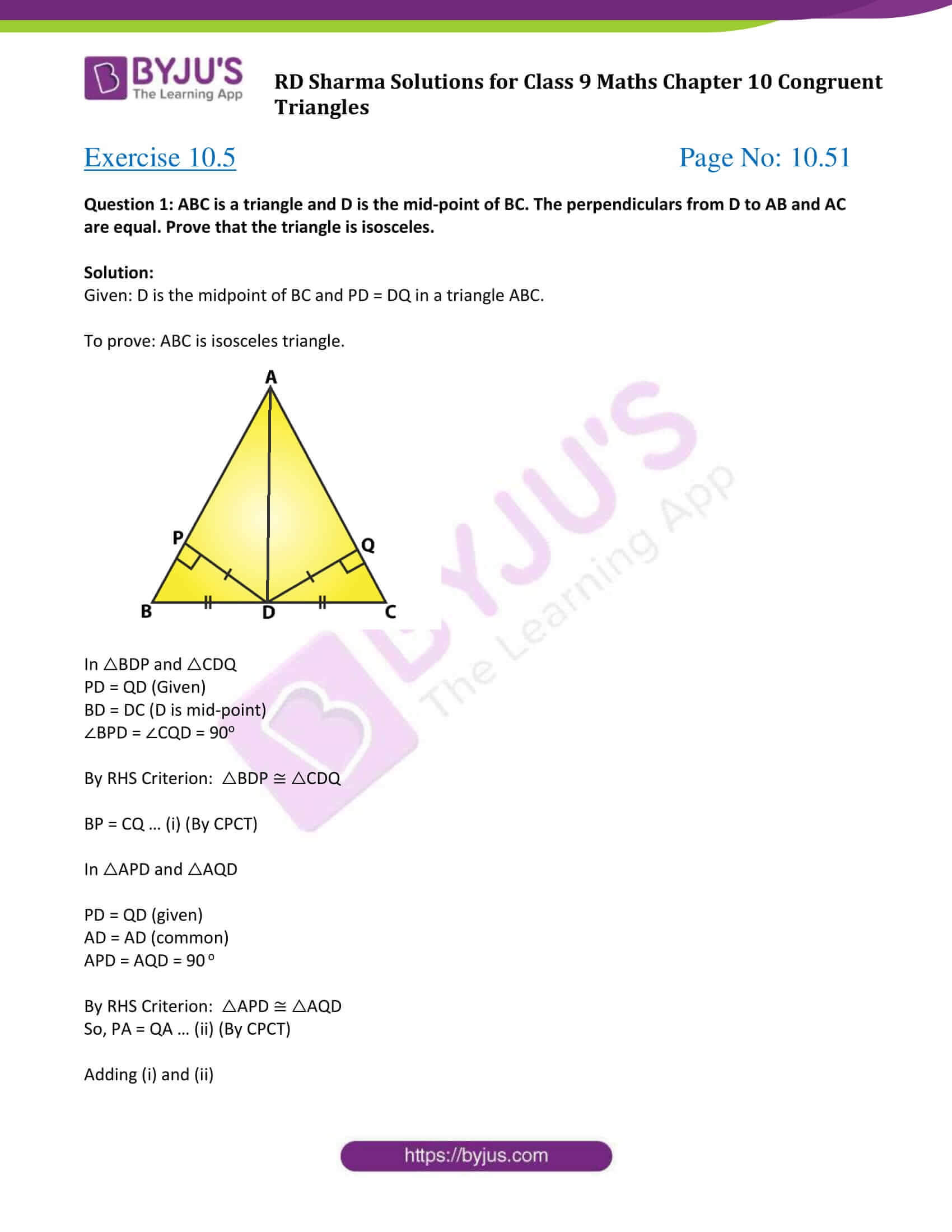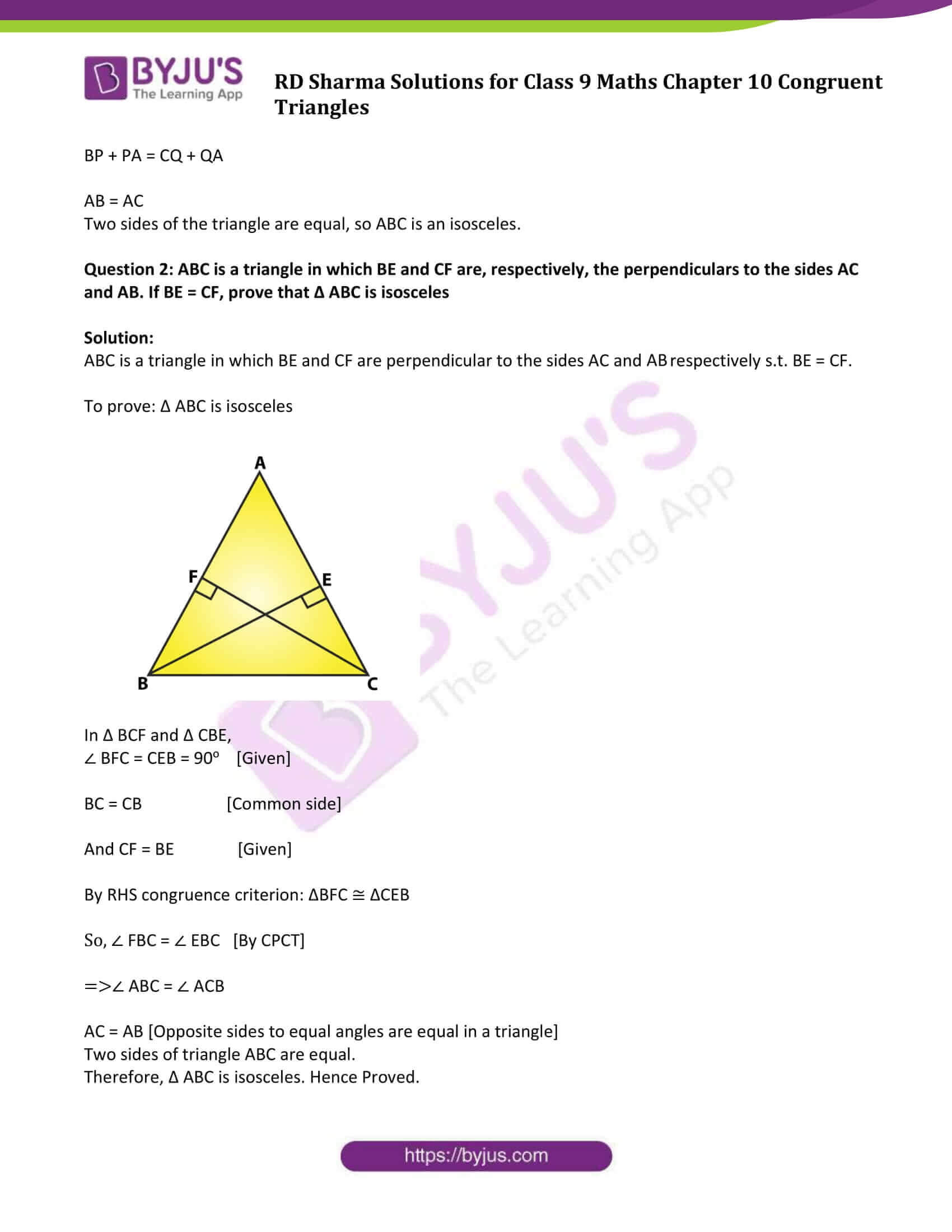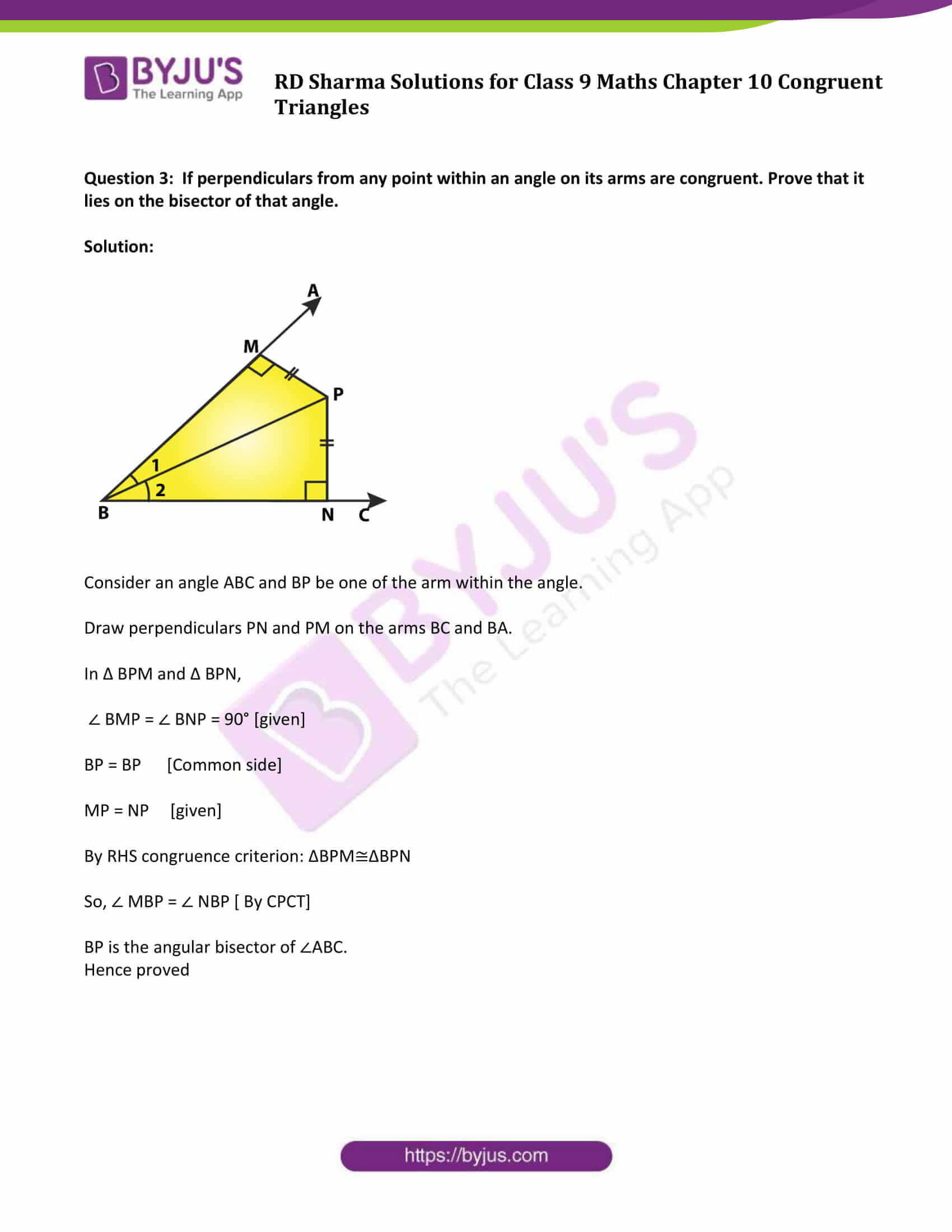### Access Answers to Maths RD Sharma Solutions for Class 9 Chapter 10 Congruent Triangles Exercise 10.5 Page number 10.51

Question 1: ABC is a triangle and D is the mid-point of BC. The perpendiculars from D to AB and AC are equal. Prove that the triangle is isosceles.

Solution:

Given: D is the midpoint of BC and PD = DQ in a triangle ABC.

To prove: ABC is isosceles triangle.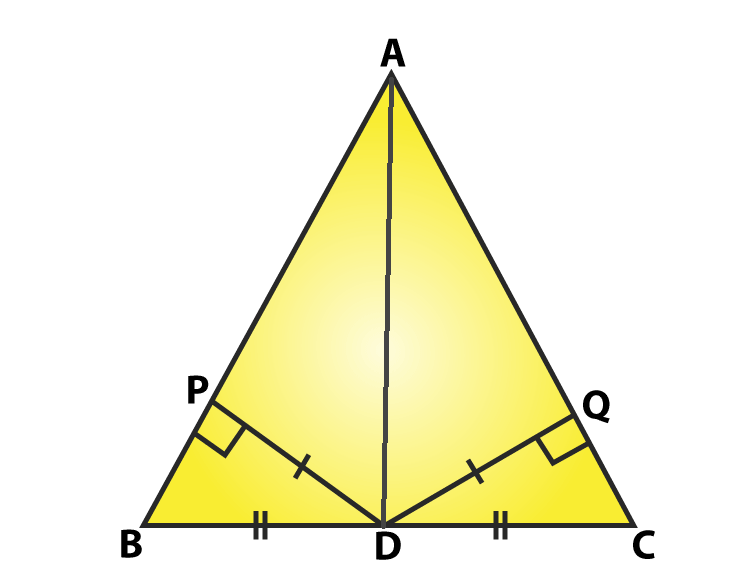In △BDP and △CDQ

PD = QD (Given)

BD = DC (D is mid-point)

∠BPD = ∠CQD = 90o

By RHS Criterion: △BDP ≅ △CDQ

BP = CQ … (i) (By CPCT)

In △APD and △AQD

PD = QD (given)

APD = AQD = 90 o

By RHS Criterion: △APD ≅ △AQD

So, PA = QA … (ii) (By CPCT)

Adding (i) and (ii)

BP + PA = CQ + QA

AB = AC

Two sides of the triangle are equal, so ABC is an isosceles.

Question 2: ABC is a triangle in which BE and CF are, respectively, the perpendiculars to the sides AC and AB. If BE = CF, prove that Δ ABC is isosceles

Solution:

ABC is a triangle in which BE and CF are perpendicular to the sides AC and AB respectively s.t. BE = CF.

To prove: Δ ABC is isosceles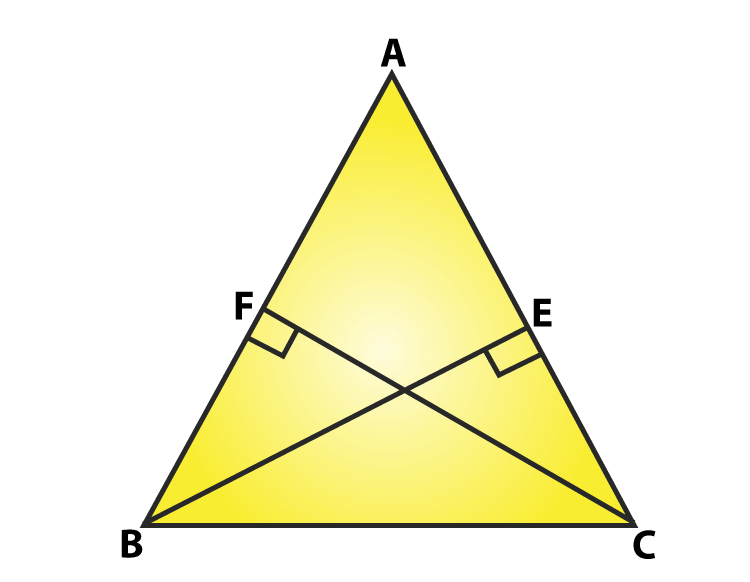In Δ BCF and Δ CBE,

∠ BFC = CEB = 90o [Given]

BC = CB [Common side]

And CF = BE [Given]

By RHS congruence criterion: ΔBFC ≅ ΔCEB

So, ∠ FBC = ∠ EBC [By CPCT]

⇒∠ ABC = ∠ ACB

AC = AB [Opposite sides to equal angles are equal in a triangle]

Two sides of triangle ABC are equal.

Therefore, Δ ABC is isosceles. Hence Proved.

Question 3: If perpendiculars from any point within an angle on its arms are congruent. Prove that it lies on the bisector of that angle.

Solution: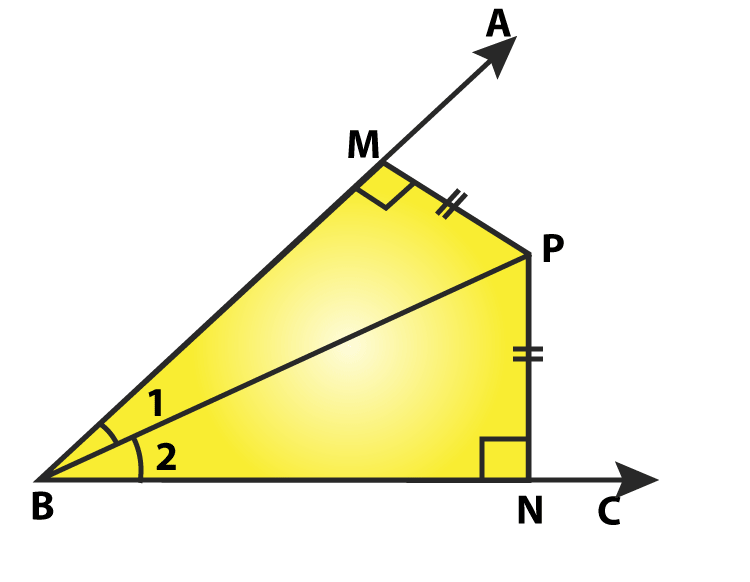Consider an angle ABC and BP be one of the arm within the angle.

Draw perpendiculars PN and PM on the arms BC and BA.

In Δ BPM and Δ BPN,

∠ BMP = ∠ BNP = 90° [given]

BP = BP [Common side]

MP = NP [given]

By RHS congruence criterion: ΔBPM≅ΔBPN

So, ∠ MBP = ∠ NBP [ By CPCT]

BP is the angular bisector of ∠ABC.

Hence proved

## RD Sharma Solutions for Class 9 Maths Chapter 10 Congruent Triangles Exercise 10.5

RD Sharma Solutions Class 9 Maths Chapter 10 Congruent Triangles Exercise 10.5 is based on the topic- Congruence Criterion – Right angle- Hypotenuse-Side. According to the criteria, two triangles are congruent if the hypotenuse and one side of one triangle are respectively equal to the hypotenuse and one side of the other triangle.